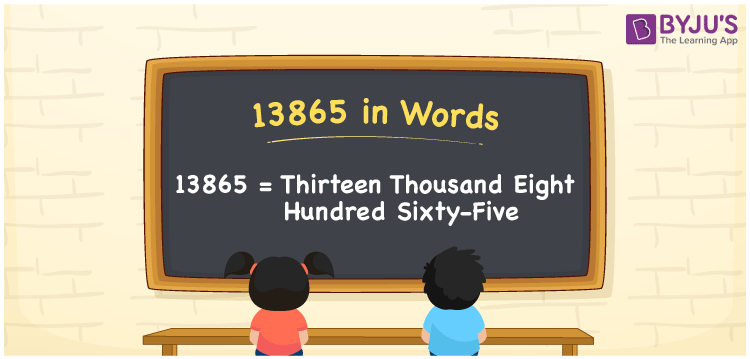# 13865 in Words

13865 in words is written as Thirteen thousand eight hundred sixty-five. In both the International System of Numerals and the Indian System of Numerals, 13865 is written as Thirteen thousand eight hundred sixty-five. The number 13865 is a Cardinal Number as it denotes some quantity. For example, “that mobile phone costs 13865 rupees”.

 13865 in Words Thirteen thousand eight hundred sixty-five Thirteen thousand eight hundred sixty-five in Number 13865

## 13865 in English Words

13865 in English words is read as “Thirteen thousand eight hundred sixty-five”.## How to Write 13865 in Words?

To write 13865 in words, we shall use the place value chart. In the place value chart, put 1 in the ten thousands, 3 in the thousands, 8 in the hundreds, 6 in the tens and 5 in the ones. Let us make a place value chart to write the number 13865 in words.

 Ten Thousands Thousands Hundreds Tens Ones 1 3 8 6 5

Thus, we can write the expanded form as

1 × Ten Thousand + 3 × Thousand + 8 × Hundred + 6 × Ten + 5 × One

= 1 × 10000 + 3 × 1000 + 8 × 100 + 6 × 10 + 5 × 1

= 10000 + 3000 + 800 + 60 + 5

= 13865

= Thirteen thousand eight hundred sixty-five.

13865 is a natural number, the successor of 13864 and the predecessor of 13866.

13865 in words – Thirteen thousand eight hundred sixty-five

• Is 13865 an odd number? – Yes
• Is 13865 an even number? – No
• Is 13865 a perfect square number? – No
• Is 13865 a perfect cube number? – No
• Is 13865 a prime number? – No
• Is 13865 a composite number? – Yes

## Frequently Asked Questions on 13865 in Words

Q1

### How to write 13865 in words?

13865 in words is written as Thirteen thousand eight hundred sixty-five.
Q2

### How to write 13865 in the International and Indian System of Numerals?

In both, the system of numerals, 13865 in words, is written as Thirteen thousand eight hundred sixty-five.
Q3

### How to write 13865 in a place value chart?

In the place value chart, write 1 in the ten thousands, 3 in the thousands, 8 in the hundreds, 6 in the tens and 5 in the ones, respectively.